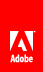# Operators

The following table provides the meaning of each operator that is available in expressions.

Operator

Meaning

and

logical AND

or

logical OR

>

greater than

>=

greater than or equal to

<

less than

<=

less than or equal to

!=

does not equal

=

equals

div

division

mod

modulus

*

multiply

+